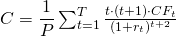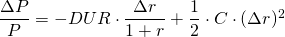# Convexity

Changes in bond values can be approximated by using duration. However, when interest rate changes are quite large, the quality of this approximation deteriorates. In case of severe changes, the approximation in bond value changes can be improved by using convexity.

## Calculation and meaning

The convexity of a bond (C) measures how curved the relationship is between the bond’s price (P) and interest rate changes (Δr). In mathematical terms, it is the second derivative of this relationship, or the first derivative of the duration. The latter means that convexity measures how much the duration changes when the interest rates shift.## Bond value, duration and convexity

In order to calculate the bond value percentage change, the formula now incorporates also convexity (C). Just as before, the duration is used to calculate an initial approximation of the price change (ΔP) which is then further refined by the convexity part. The approximation only improves to a minor extent in case of small interest rate changes. In case of major shift, the convexity adjusted approximation provides major improvements.Suppose a bond has maturity 5 years, with a principle of 1000, pays a coupon of 5% annually and the market rate equals 6.5%. If the market discount rates suddenly decrease to 3% the bond value would truly increase by 153,93 or 16.42%. The duration approximation would indicate an adjustment of 148.84 or 14.88% which is too low. The convexity adjusted formula indicates a change of 152.86 or 16.30% which is much closer to the true change. In case the market discount rat increases to 10% annually, the bond value would truly decrease by 93.25 or 9.95%. The duration overestimates this: a drop of 99.69 or 10.63% is indicated. The convexity approximation on the other hand gives a decrease of 92.90 or 9.91%, again much closer to the real drop.

## Summary

Convexity enables getting better bond price change approximations when interest rates change heavily. It is used as an additional risk metric and measures the change in duration when interest rates shift.